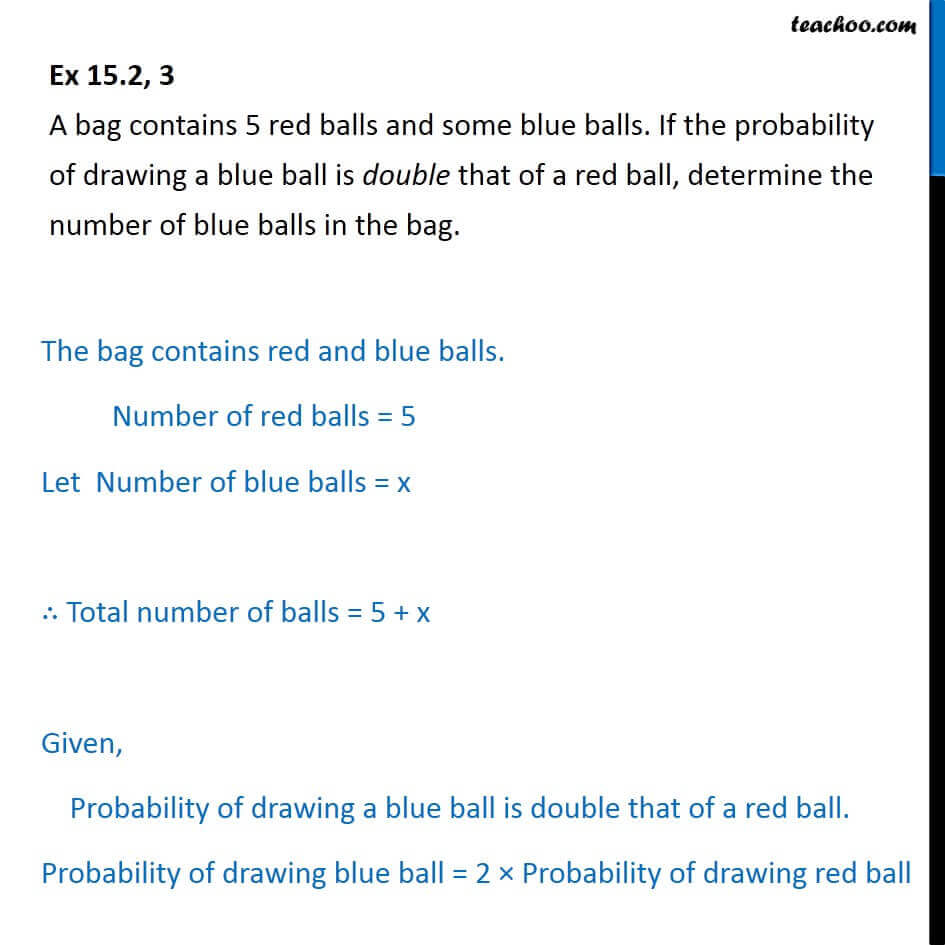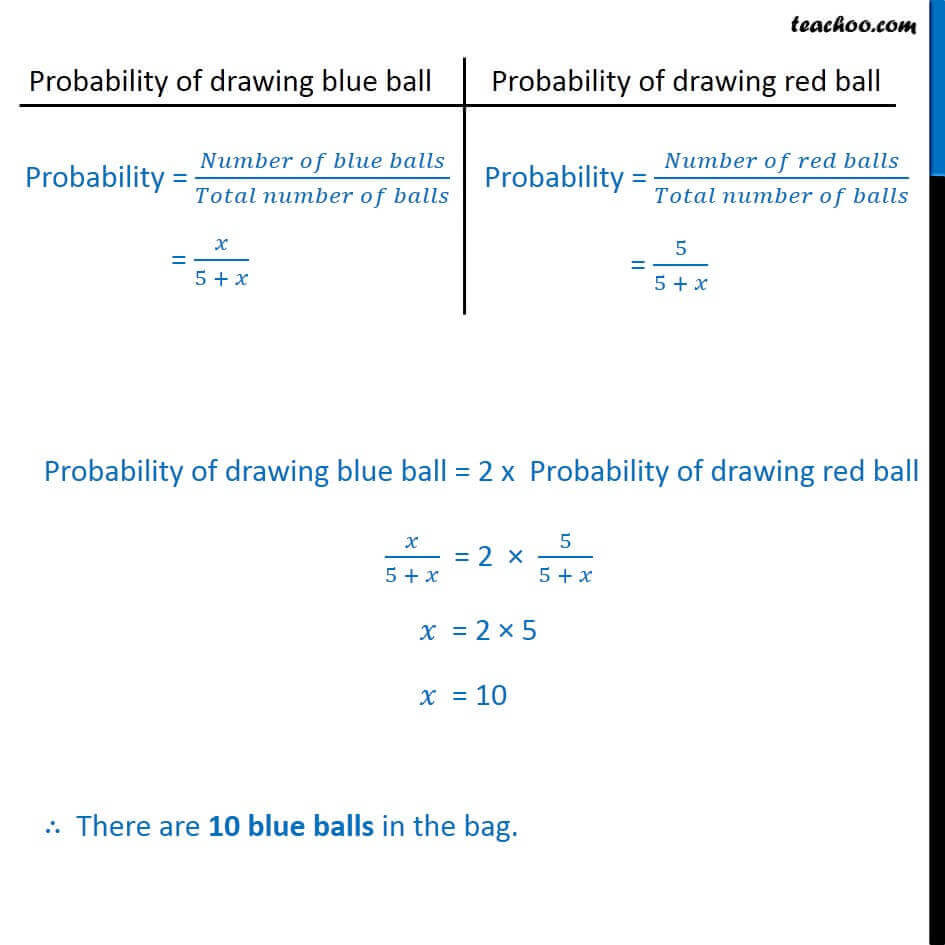Important Probability Questions

Chapter 14 Class 10 Probability
Serial order wiseLearn in your speed, with individual attention - Teachoo Maths 1-on-1 Class

### Transcript

Question 3 A bag contains 5 red balls and some blue balls. If the probability of drawing a blue ball is double that of a red ball, determine the number of blue balls in the bag.The bag contains red and blue balls. Number of red balls = 5 Let Number of blue balls = x ∴ Total number of balls = 5 + x Given, Probability of drawing a blue ball is double that of a red ball. Probability of drawing blue ball = 2 × Probability of drawing red ball Probability = (𝑁𝑢𝑚𝑏𝑒𝑟 𝑜𝑓 𝑏𝑙𝑢𝑒 𝑏𝑎𝑙𝑙𝑠)/(𝑇𝑜𝑡𝑎𝑙 𝑛𝑢𝑚𝑏𝑒𝑟 𝑜𝑓 𝑏𝑎𝑙𝑙𝑠) = 𝑥/(5 + 𝑥) Probability = (𝑁𝑢𝑚𝑏𝑒𝑟 𝑜𝑓 𝑟𝑒𝑑 𝑏𝑎𝑙𝑙𝑠)/(𝑇𝑜𝑡𝑎𝑙 𝑛𝑢𝑚𝑏𝑒𝑟 𝑜𝑓 𝑏𝑎𝑙𝑙𝑠) = 5/(5 + 𝑥) Probability of drawing blue ball = 2 x Probability of drawing red ball 𝑥/(5 + 𝑥) = 2 × 5/(5 + 𝑥) 𝑥 = 2 × 5 𝑥 = 10 ∴ There are 10 blue balls in the bag.